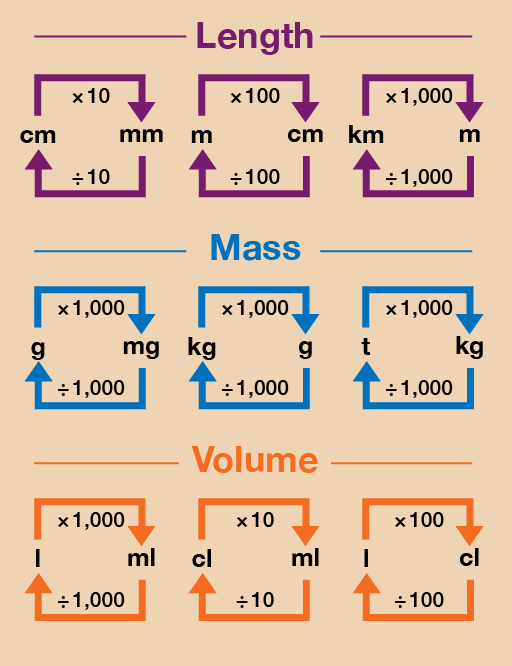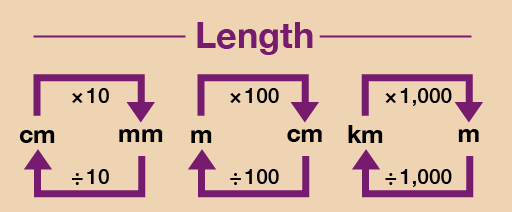Everyday maths 1

Start this free course now. Just create an account and sign in. Enrol and complete the course for a free statement of participation or digital badge if available.

Free course

# 1.1 Changing units

Sometimes you will need to change between millimetres and centimetres, or centimetres and metres. For example, you might need to do this if you were fitting a kitchen or measuring a piece of furniture.

The diagram below shows you how to convert between metric units if you’re calculating any of the following:

• length, which is a measurement of how long something is
• mass (sometimes referred to as weight), which is a measurement of how heavy something is
• volume (sometimes referred to as capacity), which is a measurement of how much space something takes up.Figure 8 A conversion chart for length, mass and volume

Starting with the smallest, metric measures of length are in millimetres, centimetres and metres. These three measurements are all related:

10 millimetres (or mm, for short) = 1 centimetre (cm)

100 cm = 1 metre (m).

Please take a look at the example below on how to carry out simple metric conversions.

Hint: 10 millimetres = 1 centimetre, 100 centimetres = 1 metre

## Example: Making Christmas cards

You are making Christmas cards for a craft stall. You want to add a bow, which takes 10 cm of ribbon, to each card. You plan to make 50 cards. How many metres of ribbon do you need?

### Method

First you need to work out how many centimetres of ribbon you need:

• 10 × 50 = 500 cm

Notice that the question asks how many metres of ribbon you need, rather than centimetres. So you need to divide 500 cm by 100 to find out the answer in metres:

• 500 ÷ 100 = 5 m

Do you remember the metric conversion diagram at the start of this session?Figure 9 A conversion chart for length

Now try the following activity. Remember to check your answers once you have completed the questions.

## Activity 2: Measuring lengths

1. You are fitting kitchen cabinets. The gap for the last cabinet is 80 cm. The sizes of the cabinets are shown in millimetres. Which size should you look for?
2. Thirty children in a class each need 20 cm of string for a project. How many metres of string will they use all together?
3. You want to buy 30 cm of fabric. The fabric is sold by the metre. What should you ask for?

You will have found it useful to refer to the metric conversion diagram for this activity.

1. To convert from centimetres to millimetres, you need to multiply the figure in centimetres by 10. The size is 80 cm, so the answer is:
• 80 × 10 = 800 mm
2. Thirty children each need 20 cm of string. To find the total in centimetres you would do the following:
• 30 × 20 = 600 cm

However, the question asked for how much string is needed in metres, not centimetres. To convert from centimetres to metres, you need to divide the figure in centimetres by 100. So if you need 600 cm, the answer is:

• 600 ÷ 100 = 6 m
1. To convert from centimetres to metres, you need to divide the figure in centimetres by 100. The length of fabric you need size is 30 cm, so the answer is:
• 30 ÷ 100 = 0.3 m

Now have a go at the following quickfire activity, using the conversion chart above if needed.

## Activity 3: Converting lengths

What are these lengths in another unit of measurement? Select the correct answers from the list of options below.

1. 20 mm = ? cm

a.

200 cm

b.

2 cm

c.

0.2 cm

1. 450 mm = ? m

a.

45 m

b.

4.5 m

c.

0.45 m

1. 0.5 cm = ? mm

a.

5 mm

b.

15 mm

c.

50 mm

1. 400 cm = ? m

a.

0.4 m

b.

4 m

c.

40 m

## Summary

In this section you have looked at measuring and calculating length. You have used different metric measurements, such as kilometres, metres and centimetres. You can now:

• measure and understand the sizes of objects
• understand different units of measurement.
FSM_1